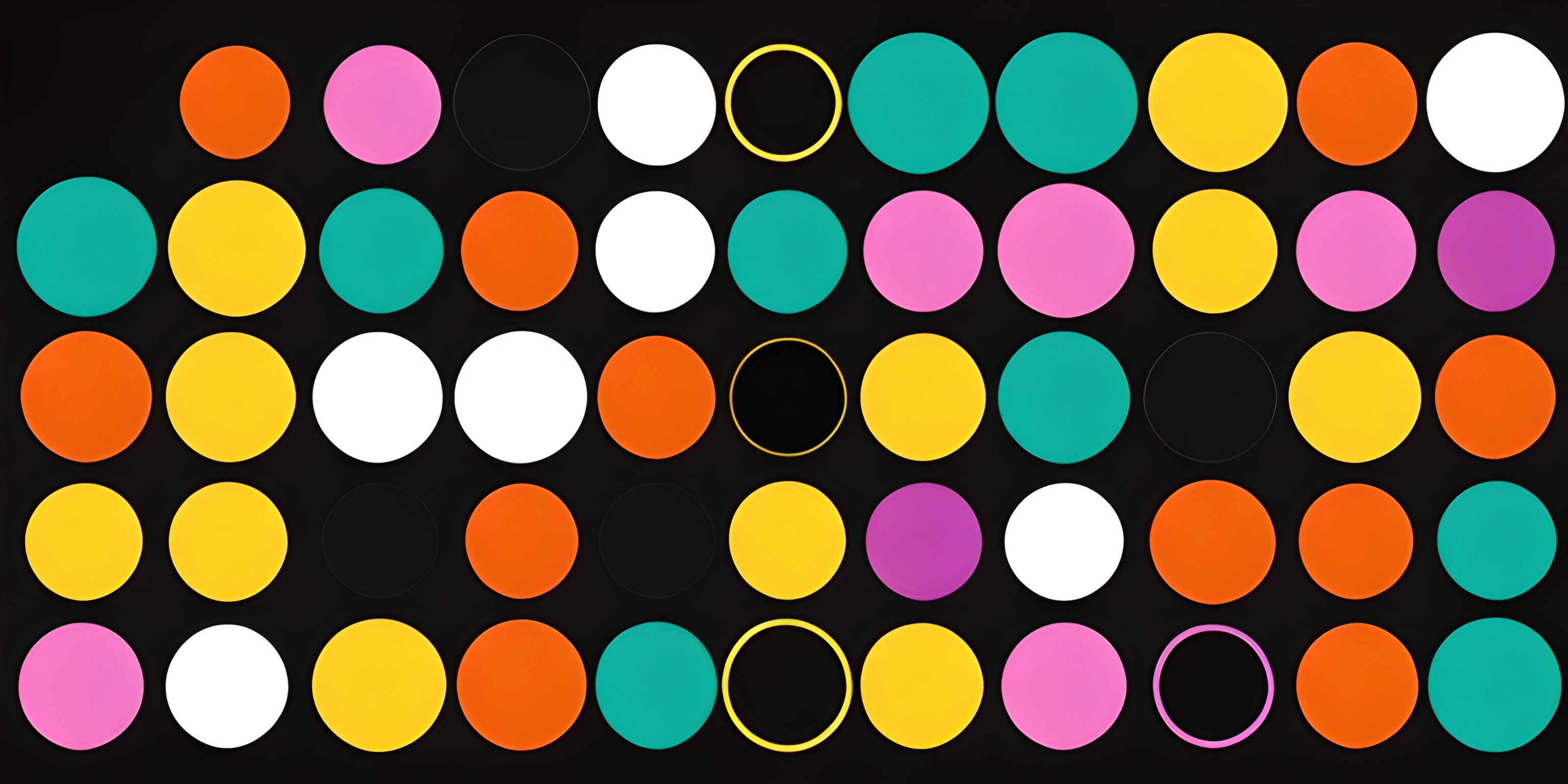# P5.js Animation EssentialsNote: this page has been created with the use of AI. Please take caution, and note that the content of this page does not necessarily reflect the opinion of Cratecode.

Creating animations can be a fun and rewarding way to bring your digital art to life. P5.js, a JavaScript library specifically designed for digital art and animation, makes it easy to create interactive visuals. So buckle up, and let's dive into the wonderful world of p5.js animations!

## The Basics

P5.js animations are created using a combination of setup() and draw() functions. The `setup()` function is executed once when the program starts, while the `draw()` function runs repeatedly in a loop, updating the canvas at each iteration.

### Setting up the Canvas

The first step in creating an animation is to set up a canvas. We do this using the `createCanvas()` function within the `setup()` function. Here's an example:

``````function setup() {
createCanvas(800, 600);
}``````

This creates an 800x600 pixel canvas for our animation to play on.

### Drawing Shapes

P5.js offers a variety of shape-drawing functions such as `rect()`, `ellipse()`, and `line()`. Let's add a simple static square to our canvas using the `rect()` function in the `draw()` function:

``````function draw() {
rect(50, 50, 100, 100);
}``````

This will draw a 100x100 pixel square at (50, 50) coordinates on the canvas.

## Animating Shapes

To make our square move, we need to update its position in each iteration of the `draw()` function. We can do this by creating a variable to store the square's position and incrementing it at each frame.

``````let xPos = 50;

function draw() {
background(255); // Clear the canvas
rect(xPos, 50, 100, 100);
xPos += 1; // Increment the x position
}``````

This will make our square move to the right across the canvas. The `background(255)` line is essential for clearing the canvas on each frame, preventing the square from leaving a trail behind.

P5.js allows us to add interactivity to our animations by responding to user input like mouse clicks or key presses. For instance, let's make the square change color when the mouse is pressed:

``````let squareColor = 0;

function draw() {
background(255);
fill(squareColor);
rect(xPos, 50, 100, 100);
xPos += 1;
}

function mousePressed() {
squareColor = random(255);
}``````

Now, whenever the user clicks the mouse, the square will change to a random grayscale color.

## Combining Multiple Shapes

To create more complex animations, we can combine multiple shapes and apply transformations like rotation and scaling. Let's add a rotating triangle to our animation:

``````let angle = 0;

function draw() {
background(255);
fill(squareColor);
rect(xPos, 50, 100, 100);
xPos += 1;

push(); // Save current drawing state
translate(width / 2, height / 2); // Move to center of canvas